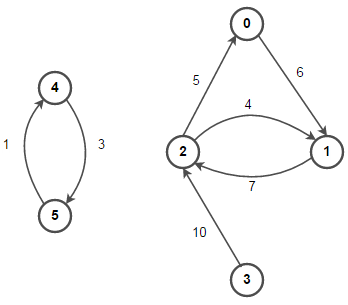# Implement Graph Data Structure in C

In this post we will see how to implement graph data structure in C using Adjacency List. This post will cover both weighted and unweighted implementation of directed and undirected graphs.

In adjacency list representation of the graph, each vertex in the graph is associated with the collection of its neighboring vertices or edges i.e every vertex stores a list of adjacent vertices.For example, for above graph below is its Adjacency List pictorial representation –## 1. Directed Graph Implementation –

Below is C implementation of a directed graph using Adjacency list:

Output:

(0 -> 1)
(1 -> 2)
(2 -> 1) (2 -> 0)
(3 -> 2)
(4 -> 5)
(5 -> 4)

As evident from above code, in a directed graph we only creates an edge from src to dest in the adjacency list. Now if the graph is undirected, we also need to create an edge from dest to src in the adjacency list as shown below:

Output:

(0 -> 2) (0 -> 1)
(1 -> 2) (1 -> 2) (1 -> 0)
(2 -> 3) (2 -> 1) (2 -> 0) (2 -> 1)
(3 -> 2)
(4 -> 5) (4 -> 5)
(5 -> 4) (5 -> 4)

## 2. Weighted Directed Graph Implementation –

In a weighted graph, each edge will have weight (or cost) associated with it as shown below:Below is C implementation of a weighted directed graph using Adjacency list. The implementation is similar to that of unweighted directed graph, except we’re also storing weight info along with every edge.

Output:

0 -> 1 (6)
1 -> 2 (7)
2 -> 1 (4) 2 -> 0 (5)
3 -> 2 (10)
4 -> 5 (1)
5 -> 4 (3)

For weighted undirected graphs, as seen before for unweighted undirected graphs, we just need to create a path from dest to src as well in the adjacency list. The complete implementation can be seen here.

Also see:(2 votes, average: 5.00 out of 5)Loading...

Please use our online compiler to post code in comments. To contribute, get in touch with us.
Like us? Please spread the word and help us grow. Happy coding 🙂